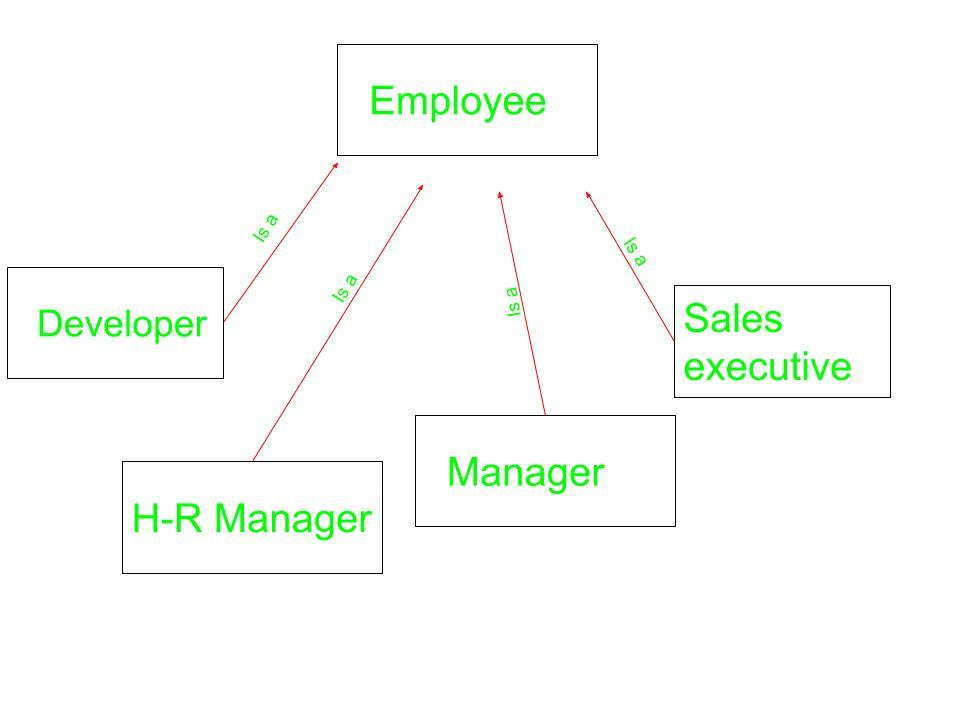GeeksforGeeks App
Open AppBrowser
Continue

## Related Articles

We can create an object of one class into another and that object will be a member of the class. This type of relationship between classes is known as containership or has_a relationship as one class contain the object of another class. And the class which contains the object and members of another class in this kind of relationship is called a container class.
The object that is part of another object is called contained object, whereas object that contains another object as its part or attribute is called container object.

Difference between containership and inheritance

Containership
-> When features of existing class are wanted inside your new class, but, not its interface
for eg->
1)computer system has a hard disk
2)car has an Engine, chassis, steering wheels.

Inheritance
-> When you want to force the new type to be the same type as the base class.
for eg->
1)computer system is an electronic device
2)Car is a vehicleEmployees can be of Different types as can be seen above. It can be a developer, an HR manager, a sales executive, and so on. Each one of them belongs to Different problem domain but the basic Characteristics of an employee are common to all.

Syntax for Containership:

```// Class that is to be contained
class first {
.
.
};

// Container class
class second {

// creating object of first
first f;
.
.
};
```

Below examples explain the Containership in C++ in a better way.

Example 1:

 `// CPP program to illustrate``// concept of Containership`` ` `#include ``using` `namespace` `std;`` ` `class` `first {``public``:``    ``void` `showf()``    ``{``        ``cout << ``"Hello from first class\n"``;``    ``}``};`` ` `// Container class``class` `second {``    ``// creating object of first``    ``first f;`` ` `public``:``    ``// constructor``    ``second()``    ``{``        ``// calling function of first class``        ``f.showf();``    ``}``};`` ` `int` `main()``{``    ``// creating object of second``    ``second s;``}`

Output:

```Hello from first class
```

Explanation:In the class second we have an object of class first. This is another type of inheritance we are witnessing. This type of inheritance is known as has_a relationship as we say that class second has an object of first class first as its member. From the object f we call the function of class first.

Example 2:

 `#include ``using` `namespace` `std;`` ` `class` `first {``public``:``    ``first()``    ``{``        ``cout << ``"Hello from first class\n"``;``    ``}``};`` ` `// Container class``class` `second {``    ``// creating object of first``    ``first f;`` ` `public``:``    ``// constructor``    ``second()``    ``{``        ``cout << ``"Hello from second class\n"``;``    ``}``};`` ` `int` `main()``{``    ``// creating object of second``    ``second s;``}`

Output:

```Hello from first class
Hello from second class
```

Explanation:In this program we have not inherited class first into class second but as we are having an object of class first as a member of class second. So when default constructor of class second is called, due to presence of object f of first class in second, default constructor of class first is called first and then default constructor of class second is called .

Example 3:

 `#include ``using` `namespace` `std;`` ` `class` `first {``private``:``    ``int` `num;`` ` `public``:``    ``void` `showf()``    ``{``        ``cout << ``"Hello from first class\n"``;``        ``cout << ``"num = "` `<< num << endl;``    ``}`` ` `    ``int``& getnum()``    ``{``        ``return` `num;``    ``}``};`` ` `// Container class``class` `second {``    ``// creating object of first``    ``first f;`` ` `public``:``    ``// constructor``    ``second()``    ``{``        ``f.getnum() = 20;``        ``f.showf();``    ``}``};`` ` `int` `main()``{``    ``// creating object of second``    ``second s;``}`

Output:

```Hello from first class
num = 20
```

Explanation:With the help of containership we can only use public member/function of the class but not protected or private. In the first class we have returned the reference with the help of getnum. Then we show it by a call to showf.

Example 4

 `#include``using` `namespace` `std;`` ` `class` `cDate``{``    ``int` `mDay,mMonth,mYear;``public``:``    ``cDate()``    ``{``        ``mDay = 10;``        ``mMonth = 11;``        ``mYear = 1999;``    ``}``    ``cDate(``int` `d,``int` `m ,``int` `y)``    ``{``        ``mDay = d;``        ``mMonth = m;``        ``mYear = y;``    ``}``    ``void` `display()``    ``{``        ``cout << ``"day"` `<< mDay << endl;``        ``cout <<``"Month"` `<< mMonth << endl;``        ``cout << ``"Year"` `<< mYear << endl;``    ``}``};``// Container class``class` `cEmployee         ``{``protected``:``    ``int` `mId;``    ``int` `mBasicSal;``    ``// Contained Object``    ``cDate mBdate;       ``public``:``    ``cEmployee()``    ``{``        ``mId = 1;``        ``mBasicSal = 10000;``        ``mBdate = cDate();``    ``}``    ``cEmployee(``int``, ``int``, ``int``, ``int``, ``int``);``    ``void` `display();``};`` ` `cEmployee :: cEmployee(``int` `i, ``int` `sal, ``int` `d, ``int` `m, ``int` `y)``{``    ``mId = i;``    ``mBasicSal = sal;``    ``mBdate = cDate(d,m,y);``}``void` `cEmployee::display()``{``    ``cout << ``"Id : "` `<< mId << endl;``    ``cout << ``"Salary :"` `<

```output
Id : 1
Salary :10000
day 10
Month 11
Year 1999
Id : 2
Salary :20000
day 11
Month 11
Year 1999
```

My Personal Notes arrow_drop_up